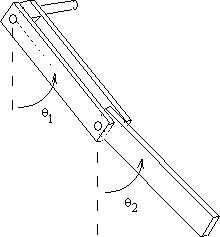## What is a Chaotic Pendulum?Figure 1: Photograph of the Chaotic Pendulum: Camera Obscura and World of Illusions, Edinburgh

A coupled, double pendulum achieves chaotic motion when non-linear initial conditions are applied; therefore, it is known as a chaotic pendulum.

A double pendulum executes simple harmonic motion when displacements from equilibrium are small. Simple harmonic motion takes place when the restoring force is directly proportional to the displacement and acts in the direction opposite to that of the displacement.

However, when large displacements are imposed, the non-linear system becomes dramatically chaotic in its motion and demonstrates that deterministic systems are not necessarily predictable.Figure 2: Drawing of a double pendulum

## How to set up the chaotic pendulum

The double pendulum must be tightly clamped in place, and this is because any unnecessary vibrations will use up the energy. This would mean that the pendulum wouldn’t swing for as long when turned using the handle. The Chaotic Pendulum at Camera Obscura is illuminated with a red, blue and green light for added effect in the dark room, as shown in Figure 1.

## How does the chaotic pendulum work?

A double pendulum consists of two physical pendulums, each can rotate a full 360° around its pivot. Each pendulum has two arms, the upper pendulum has a slightly longer arm, but both have the same mass. In a simple double pendulum, the mass is distributed through the length, hence the angle between the two arms is used to calculate the moment of inertia. The pendulum undergoes chaotic motion, generating regions where the momentum exceeds the weight plus the gravitational pull and the second pendulum rotates fully around its pivot.

The regions are generated dependent on the transfer of the potential energy to kinetic energy and vice-versa in the rotation before it. Math theories like Lagrangian formulation for the dynamics can be used to calculate these.

The system is known to be chaotic as the path is randomised every time one rotation is achieved. The pendulums rotate clockwise and anti-clockwise in the most unexpected ways, changing direction or suddenly increasing speed when you least expect it, therefore the probability of predicting its path is very low.

Recently Google has come up with a new quantum computer, said to predict these random movements.

## What is a Quantum Computer?

Classical computers store information on a binary system which frequently uses ‘0’ and ‘1’ as bits, a quantum computer performs calculations based on the laws of quantum mechanics. The foundation of quantum mechanics is that subatomic particles can exist in more than one state at any time. Using this ‘superposition nature’ of subatomic particles as a highly efficient way to store and process information, the quantum computer has managed to transform its commutating process into the one that enables parallelism.

According to physicists, this parallelism gives quantum computers the potential to conduct a million computations at once while the classical computers can only work with a limited number of binary numbers each time, which is much fewer than that of the quantum computer.

As a result, quantum computers have the potential to predict the chaotic pattern of the pendulum such as the one we have in Camera Obscura and World of Illusions.

Our Chaotic Pendulum can be found on the second floor in our Bewilderworld exhibition, next to the popular Mirror Maze and Vortex Tunnel. Give it a spin and see what happens!

## Separate Information about Quantum Computers

Apart from its potential use in predicting the trajectory of the pendulum, the main implication of quantum computer lies at the heart of code decryption due to its extraordinary computation ability.

“A quantum computer using Shor’s algorithm could achieve the same feat in just 10 seconds, with a modest 1 million operations per second.” Said Andreas Baumhof, World Economic Forum.

Even with their exceptional capability, it should be noted that quantum computers are not likely to entirely replace classical computers. For example, it has been tested that currently, classical computers are better at dealing with everyday activities such as spreadsheets and email due to the different calculation methods each type of computer uses.

“My best guess would be that: the quantum computers would be used as an accelerator for problems that are very hard for classical machines,” Said Professor Mikhail Lukin, co-director of Harvard’s Quantum initiative.